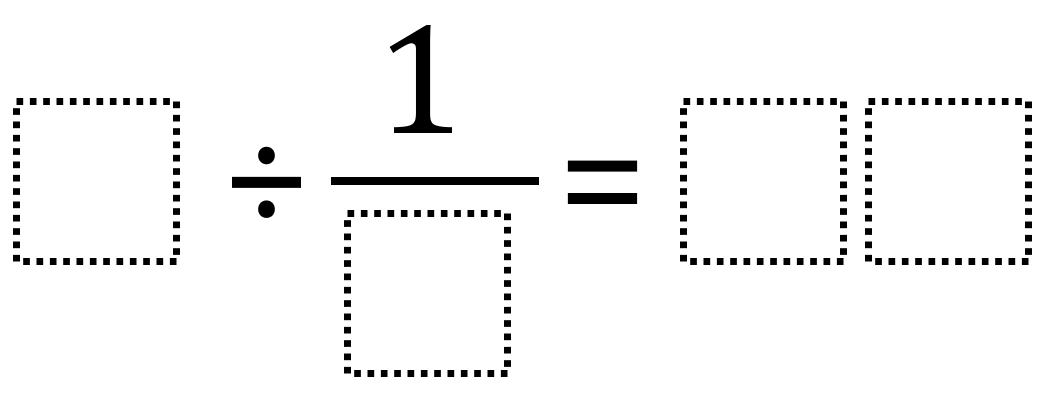# Dividing Fractions 3

Directions: Using the digits 1 through 9, at most one time each, place a digit in each box to create two true equations: one where the quotient is greater than 40 and one where it’s less than 40. You may reuse the same digits for each of the equations.### Hint

How can dividing by a single digit by a fraction result in a two-digit quotient?

There are many possible answers including 9 ÷ 1/3 = 27 and 9 ÷ 1/6 = 54.

Source: Robert Kaplinsky

## Add Fractions with Decimal Sums

Directions: Using the digits 1 to 9 at most one time each, place a digit …

### One comment

1.Less : 3 divided by 1/2 = 6
More : 9 divided by 1/8 = 72